Publication date: 11/29/2021

## Control Limits for XBar and R Charts

JMP generates control limits for XBar and R charts using the following formulas:

LCL for XBar chart =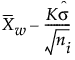UCL for XBar chart =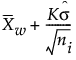LCL for R chart =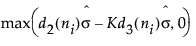UCL for R chart =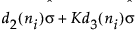Center line for R chart: By default, the center line for the ith subgroup indicates an estimate of the expected value of Ri. This value is computed as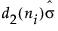, where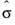is an estimate of σ.

The standard deviation for XBar and R charts is estimated using the following formula: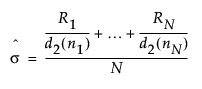where: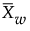= weighted average of subgroup means

K = the sigma multiplier and is set to 3 by default

σ = process standard deviation

ni = sample size of ith subgroup

d2(n) is the expected value of the range of n independent normally distributed variables with unit standard deviation

d3(n) is the standard deviation of the range of n independent normally distributed variables with unit standard deviation

Ri is the range of ith subgroup

N is the number of subgroups for which ni ≥ 2.